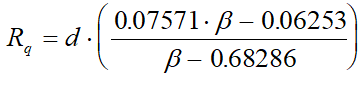# Calculation of Differential Range - Intergraph Smart Instrumentation - 13.1 - Reference - Hexagon PPM

## Integraph Smart Instrumentation Sizing Equations

Language
English (United States)
Product
Intergraph Smart Instrumentation
Search by Category
Reference
Smart Instrumentation Version
13.1
1. Calculate internal pipe diameter at flow temperature: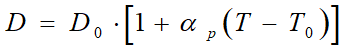2. Calculate correction factor for steam quality (for liquid, water, and gas, Fs=1):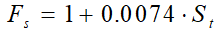3. Calculate orifice diameter at flow temperature.

1. For Lo-Loss tube, Nozzles, and Venturi tubes: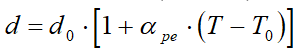2. For other orifices: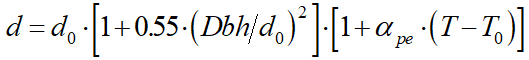4. Calculate pipe Reynolds number:

*W[kg/s]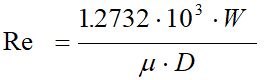5. Calculate b -ratio.

1. For all Flow meters except Segmental orifices: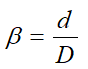2. Segmental orifices.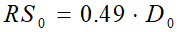2. Calculate b -ratio: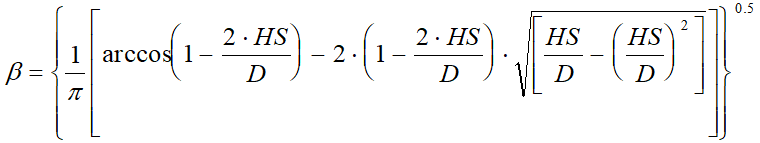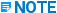HS is calculated with the help of HS0 in accordance with step 3b.

6. Calculate Discharge coefficient, see Appendix.

7. First estimate for Differential range: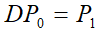8. Calculate gas expansion factor (for liquid and water, Eps=1).

1. For square edge orifice with 2½D and 8D pipe taps: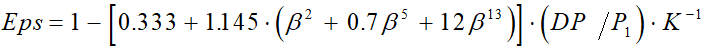2. For Lo-LOss tube, Venturi tubes and Nozzles: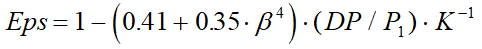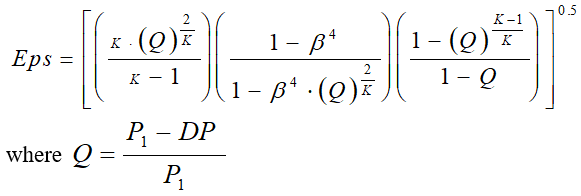3. For eccentric orifices: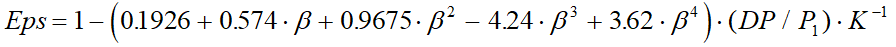4. For square-edge orifices specified in ISO 5167 - 2003 (flange tappings, corner tappings, and radius tappings):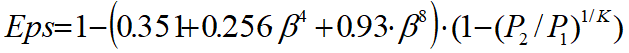5. For other Flow meters:9. Calculate new Differential range:

*W[kg/s]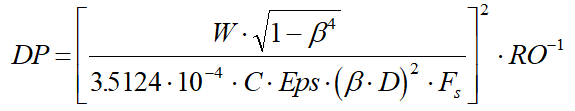10. For liquid and water, got to step 13.

11. Calculate the relative change in differential range: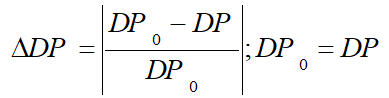12. Repeat steps 8 to 11 until the relative change in the differential range is less than 0.0001.

13. For quarter of circle (Quadrant Edge) orifice, calculate radius of upstream profile: